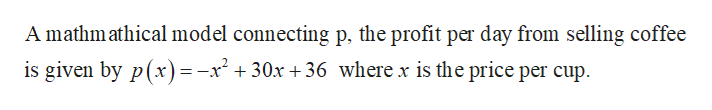# David owns a cafe. A mathmathical model connecting p, the profit per day from selling coffee (in dollars) and x, the price per cup (in dollars) is p(x)=-x2+30x+36Find the profit per day if the price per cup of coffee is \$1.90. Round to the nearest dollar.

Question
2 views

David owns a cafe. A mathmathical model connecting p, the profit per day from selling coffee (in dollars) and x, the price per cup (in dollars) is p(x)=-x2+30x+36

Find the profit per day if the price per cup of coffee is \$1.90. Round to the nearest dollar.

check_circle

Step 1help_outlineImage TranscriptioncloseA mathm athical model connecting p, the profit per day from selling coffee is given by p(x) = -x² + 30x +36 where x is the price per cup. fullscreen

### Want to see the full answer?

See Solution

#### Want to see this answer and more?

Solutions are written by subject experts who are available 24/7. Questions are typically answered within 1 hour.*

See Solution
*Response times may vary by subject and question.
Tagged in

### Other